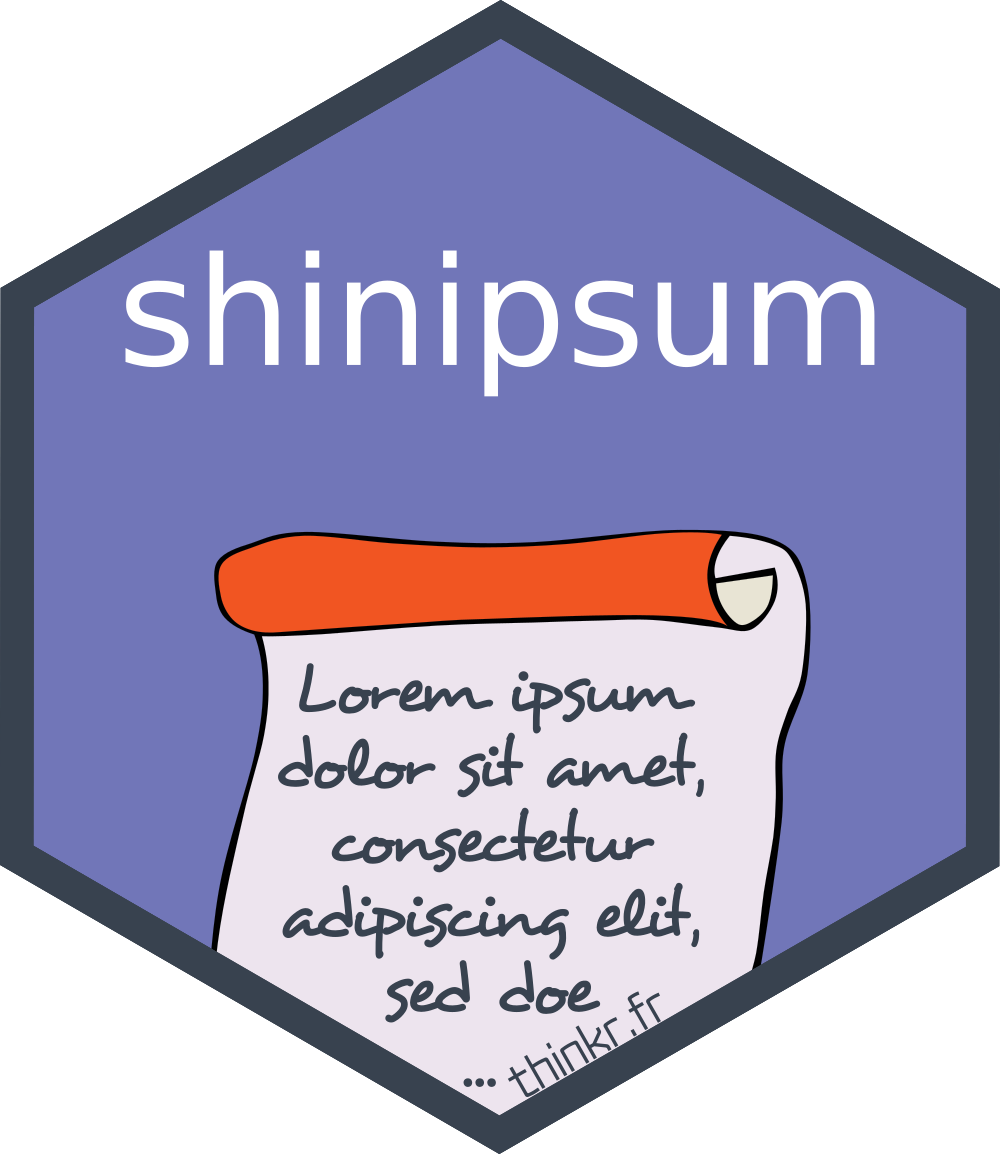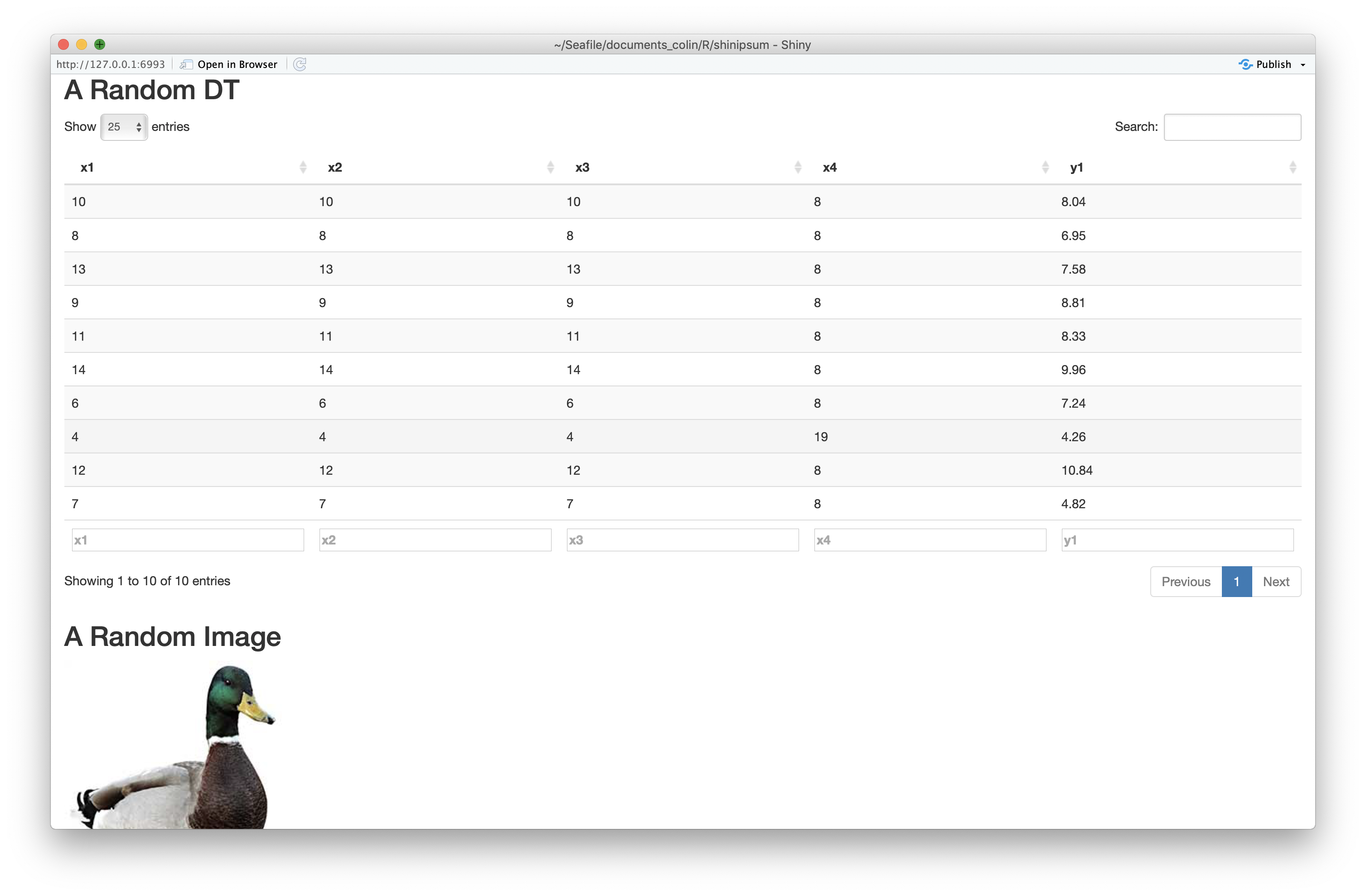shinipsum

The goal of {shinipsum} is to provide random shiny elements for easiest shiny app prototyping, so that you can focus on building the frontend before building the backend.

The full documentation is in the {pkgdown} site: https://thinkr-open.github.io/shinipsum/

Installation

You can install the dev version of shinipsum from GitHub with:

remotes::install_github("Thinkr-open/shinipsum")

Demo

Available examples:

library(shinipsum)
ipsum_examples()
#>  "01_navbar.R"

You can run {shinipsum} demos with:

shiny::runApp(
ipsum_examples("01_navbar.R")
)

Available ipsums :

Note: {shinipsum} only load functions which are necessary to its internal job. If you want to customise an output or to use a renderXX / XXOutput, you’ll need to explicitely load the packages needed (for example, if you want to customise a dygraph, a ggplot, or use ggplotly).

DataTable

random_DT takes 4 args :

• nrow & ncol: number of row and columns of the table
• type : random, numeric, character, numchar - the type of the columns
• ... : args to be passed to DT::datatable

Image

random_image returns a random image.

Plot

random_ggplot takes one arg :

• type : Can be any of “random”, “point”, “bar”, “boxplot”,“col”, “tile”, “line”, “bin2d”, “contour”, “density”, “density_2d”, “dotplot”, “hex”, “freqpoly”, “histogram”, “ribbon”, “raster”, “tile”, “violin” and defines the geom of the ggplot. Default is “random”, and chooses a random geom for you.

Default theme is minimal.

As the return object is a ggplot, it can be enhanced like any other ggplot with +.

library(ggplot2)
random_ggplot(type = "col") +
labs(title = "Random plot") +
theme_bw()

random_ggplotly calls the ggplotly function on a random_ggplot.

Dygraph

random_dygraph returns a random dygprah. It takes one arg:

• ...: arguments which are passed to the dygraph() function.

As the return object is a dygraph, it can be enhanced like any other dygraph.

library(dygraphs)
random_dygraph()  %>%
dyRangeSelector()

Print

random_print takes one arg:

• type: can be any of "character", "numeric", "model", "table", and defines the type of print. Default is "character".

Table

random_table takes three args : nrow, ncols and type. See random_DT.

Text

random_text takes one of these two args :

• nchar : lorem ipsum of nchar characters
• nwords : lorem ipsum of nwords characters

One of the two should be left NULL

Example

Here is an example of using {shinipsum} to generate a random app:

library(shiny)
library(shinipsum)
library(DT)
ui <- fluidPage(
h2("A Random DT"),
DTOutput("data_table"),
h2("A Random Image"),
plotOutput("image", height = "300px"),
h2("A Random Plot"),
plotOutput("plot"),
h2("A Random Print"),
verbatimTextOutput("print"),
h2("A Random Table"),
tableOutput("table"),
h2("A Random Text"),
tableOutput("text")
)

server <- function(input, output, session) {
output\$data_table <- DT::renderDT({
random_DT(10, 5)
})
output\$image <- renderImage({
random_image()
})
output\$plot <- renderPlot({
random_ggplot()
})
output\$print <- renderPrint({
random_print("model")
})
output\$table <- renderTable({
random_table(10, 5)
})
output\$text <- renderText({
random_text(nwords = 50)
})
}
shinyApp(ui, server)Please note that the ‘shinipsum’ project is released with a Contributor Code of Conduct. By contributing to this project, you agree to abide by its terms.